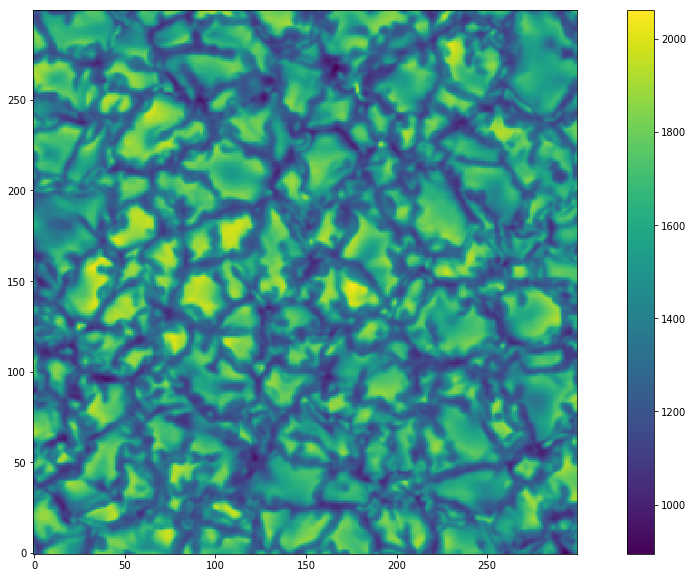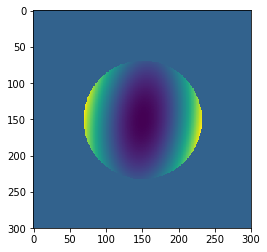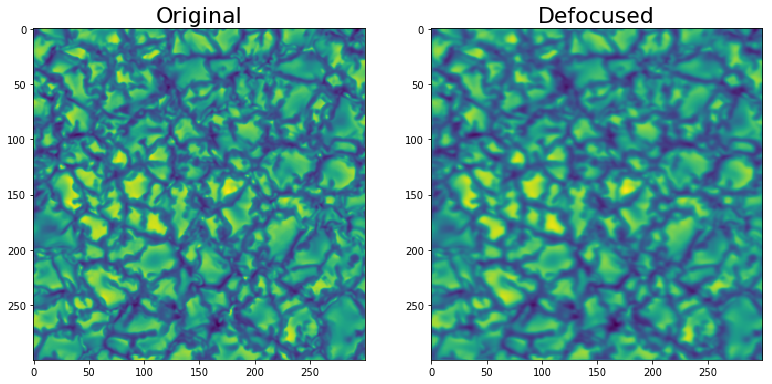# How to restore an initial scence using deconvolution

## Image Restoration - deblurring¶

### In this notebook I will show how to filter a blurred image in order to restore the initial data. I will use a simulation of granular structure (from SOPHISM simulations) that is free from optical aberrations and noise.¶

In :
```import WF_psf_mtf
from WF_psf_mtf import *
```
In :
```p = '/home/fatima/Desktop/solar_orbiter_project/codes/targets/MTF/'
data = pyfits.getdata(p+'Masi_hrt.fits')
data = data[300:600,300:600]
```
In :
```plt.figure(figsize=(18,10))
plt.imshow(data,origin='lower')
plt.colorbar()
```
Out:
`<matplotlib.colorbar.Colorbar at 0x7f02677faf50>`### OTF synthesis¶

#### We will synthesis a wavefront aberration consisting of defocus and astigmatism¶

In :
```D = 140 #diameter of the aperture
lam = 617.3*10**(-6) #wavelength of observation
pix = 0.5 #plate scale
f = 4125.3            #effective focal length
size = data.shape #size of detector in pixels
```
In :
```coefficients = np.zeros(8)
coefficients = 0
coefficients = 0
coefficients =0
coefficients = 0.5
coefficients = 0.1
coefficients = 0.4
coefficients = 0
coefficients = 0
```
In :
```rpupil = pupil_size(D,lam,pix,size)
sim_phase = center(coefficients,size,rpupil)

psf = PSF(pupil_com)
otf = OTF(psf)
mtf = MTF(otf)
```
In :
```plt.imshow(sim_phase)
```
Out:
`<matplotlib.image.AxesImage at 0x7f02679bf7d0>`## No noise¶

#### I will produce the aberrated data without adding any noise¶

In :
```fft_image = np.fft.fft2(data)
temp = fft_image*otf
defoc = np.fft.ifft2(temp).real
```
In :
```fig = plt.figure(figsize=(15,10))
im=ax.imshow(data)
im2=ax2.imshow(defoc)
ax.set_title('Original',fontsize=22)
ax2.set_title('Defocused',fontsize=22)
```
Out:
`<matplotlib.text.Text at 0x7f02678ca4d0>`#### To restore the initial scene we could use the inverse filtering, which is dividing the blurred scene with the synthesized OTF, then using the inverse Fourier Transform:¶

In :
```fft_blurred = fft2(defoc)
restored = fft_blurred/otf
restored = ifft2(restored).real
```
In :
```plt.figure(figsize=(18,10))
plt.imshow(restored,origin='lower')
plt.colorbar()
```
Out:
`<matplotlib.colorbar.Colorbar at 0x7f02535b2090>`